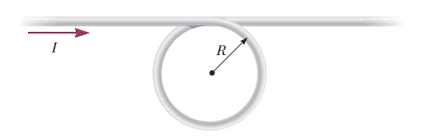# Magnetic field at the center of a loop

## Homework StatementA conductor consists of a circular loop of radius
R = 14.4 cm
and two long, straight sections as shown in the figure. The wire lies in the plane of the paper and carries a current
I = 1.60 A.
Find the magnetic field at the center of the loop.

biot-savart law
?

## The Attempt at a Solution

I know I need use biot-savart law to get the field created by the loop, which is giving me 8.77 uT. This is apparently within 10% of the correct answer.
Do i need to find the current from the straight part of the wire too (superposition)?

I'm just not sure how to do this / what equations to use to do this?

gneill
Mentor

## Homework Statement

View attachment 79319
A conductor consists of a circular loop of radius
R = 14.4 cm
and two long, straight sections as shown in the figure. The wire lies in the plane of the paper and carries a current
I = 1.60 A.
Find the magnetic field at the center of the loop.

biot-savart law
?

## The Attempt at a Solution

I know I need use biot-savart law to get the field created by the loop, which is giving me 8.77 uT. This is apparently within 10% of the correct answer.
Do i need to find the current from the straight part of the wire too (superposition)?
Yes. And superpostiion is an excellent idea.
I'm just not sure how to do this / what equations to use to do this?
You should have an expression for the magnetic field at a given distance from a current-carrying wire...

thats where i'm confused.

shouldn't i use B = (uI)/(2 pi r) ?
4pi x 10^-7 x 1.6A / 2 pi x .144m ? this gives me 2.22 uT which when added to the 8.77 is out the the correct range

Last edited:
gneill
Mentor
thats where i'm confused.

shouldn't i use B = (uI)/(2 pi r) ?
4pi x 10^-7 x 1.6A / 2 pi x .144m ? this gives me 2.22 uT which when added to the 8.77 is out the the correct range
2.22 μT looks right for the straight wire. But the loop contribution looks a bit high to me. Can you show the details of your work there?
i need to take this integral of this dont i?
No, the expression gives the field magnitude, not a differential element. The integration was already done in deriving the expression.

Ahhhh thank you! my mistake was in the calculation for the loop! i left the 4pi in place when using u/4pi

hahaah that was dumb. but thank you, i got the right answer. it's 9.20 uT!

:)